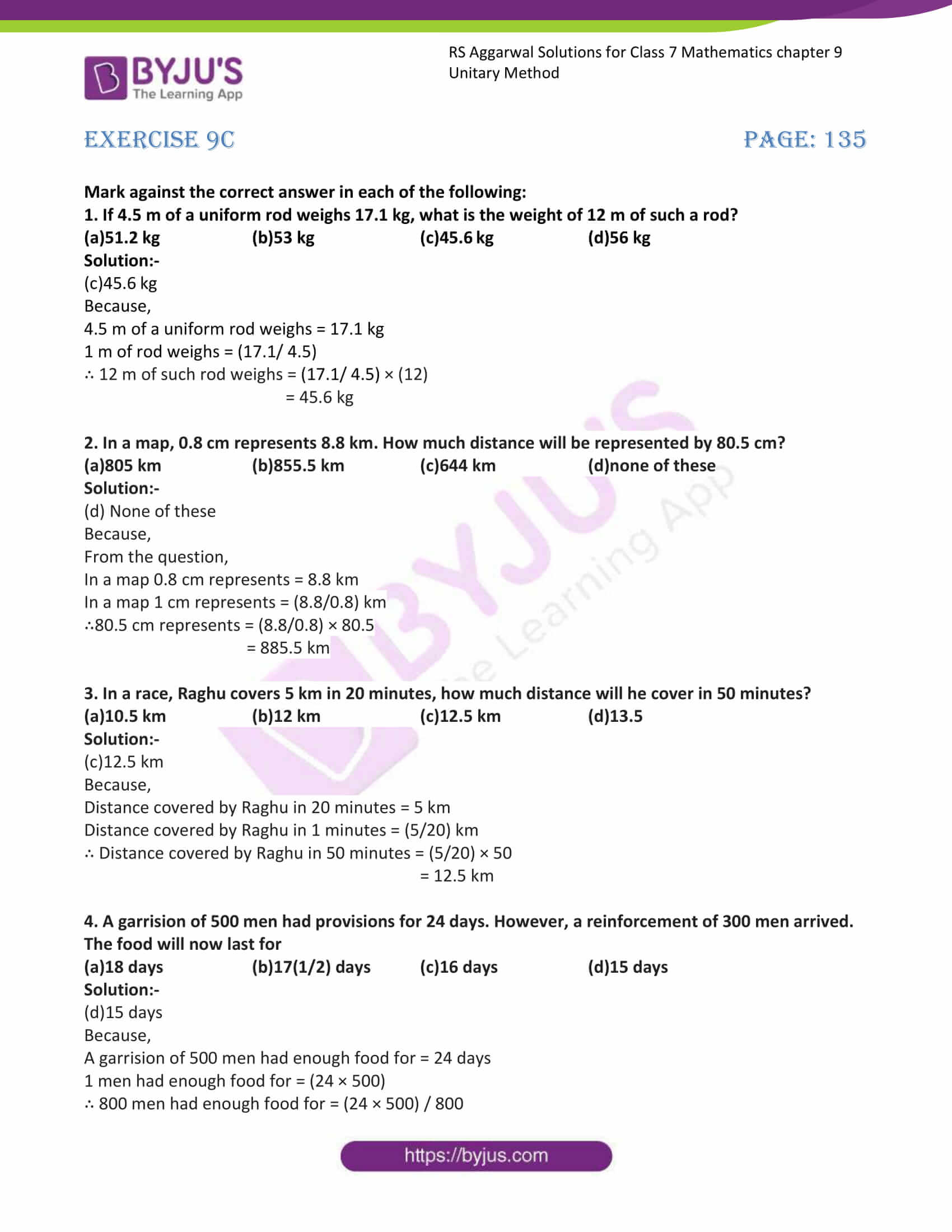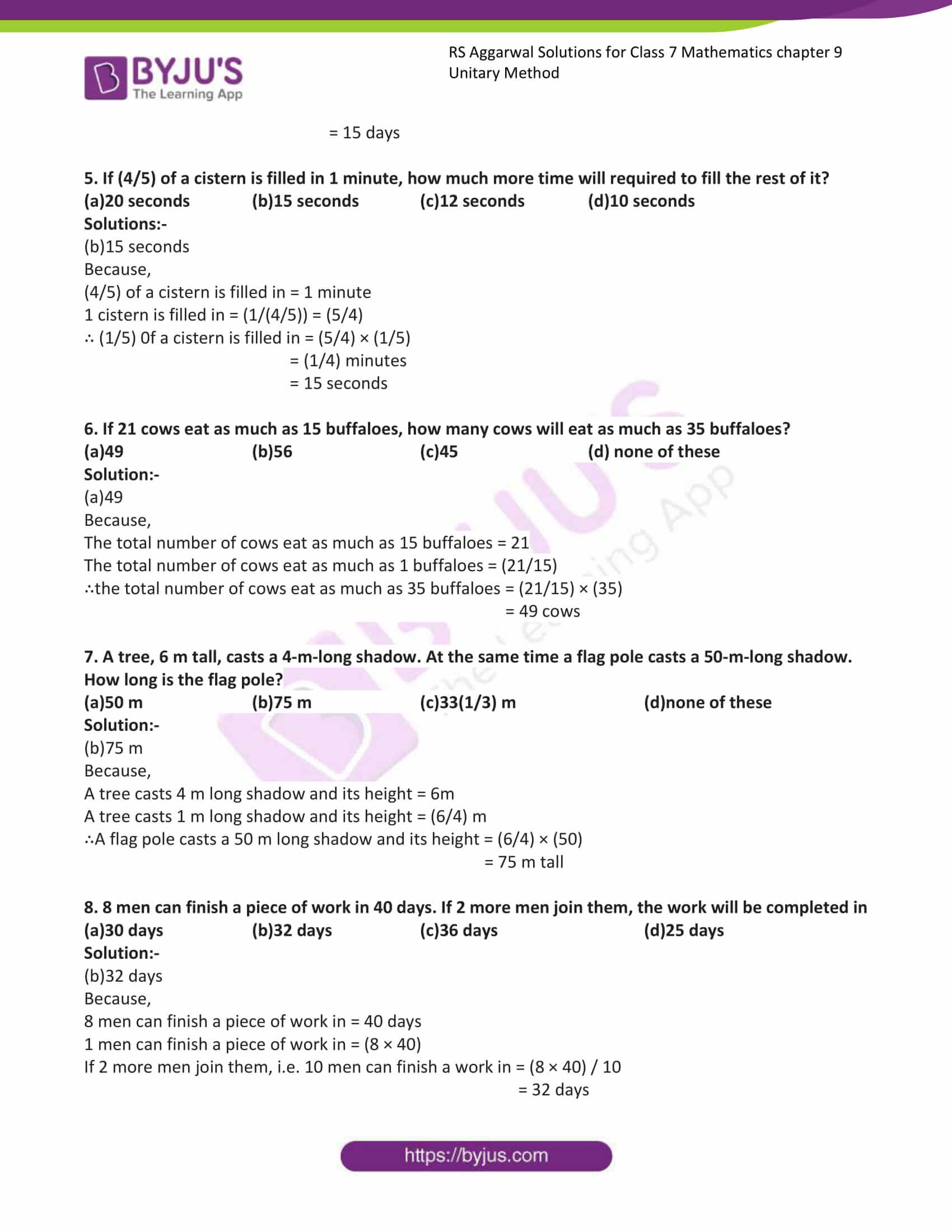# RS Aggarwal Solutions for Class 7 Maths Exercise 9C Chapter 9 Unitary Method

RS Aggarwal Solutions for the Exercise 9C of Class 7 Maths Chapter 9 has objective type questions. In this exercise, application-level questions are included to help students think out of the box to get the answers. The objective type questions in this exercise are based on all the topics covered in this chapter. Students are suggested to try solving the problems from RS Aggarwal Solutions for Class 7 Maths Chapter 9 Unitary Method.

## Download the PDF of RS Aggarwal Solutions For Class 7 Maths Chapter 9 Unitary Method – Exercise 9C### Access answers to Maths RS Aggarwal Solutions for Class 7 Chapter 9 – Unitary Method Exercise 9C

Mark against the correct answer in each of the following:

1. If 4.5 m of a uniform rod weighs 17.1 kg, what is the weight of 12 m of such a rod?

(a)51.2 kg (b)53 kg (c)45.6 kg (d)56 kg

Solution:-

(c)45.6 kg

Because,

4.5 m of a uniform rod weighs = 17.1 kg

1 m of rod weighs = (17.1/ 4.5)

∴ 12 m of such rod weighs = (17.1/ 4.5) × (12)

= 45.6 kg

2. In a map, 0.8 cm represents 8.8 km. How much distance will be represented by 80.5 cm?

(a)805 km (b)855.5 km (c)644 km (d)none of these

Solution:-

(d) None of these

Because,

From the question,

In a map 0.8 cm represents = 8.8 km

In a map 1 cm represents = (8.8/0.8) km

∴80.5 cm represents = (8.8/0.8) × 80.5

= 885.5 km

3. In a race, Raghu covers 5 km in 20 minutes, how much distance will he cover in 50 minutes?

(a)10.5 km (b)12 km (c)12.5 km (d)13.5

Solution:-

(c)12.5 km

Because,

Distance covered by Raghu in 20 minutes = 5 km

Distance covered by Raghu in 1 minutes = (5/20) km

∴ Distance covered by Raghu in 50 minutes = (5/20) × 50

= 12.5 km

4. A garrision of 500 men had provisions for 24 days. However, a reinforcement of 300 men arrived.

The food will now last for

(a)18 days (b)17(1/2) days (c)16 days (d)15 days

Solution:-

(d)15 days

Because,

A garrision of 500 men had enough food for = 24 days

1 men had enough food for = (24 × 500)

∴ 800 men had enough food for = (24 × 500) / 800

= 15 days

5. If (4/5) of a cistern is filled in 1 minute, how much more time will required to fill the rest of it?

(a)20 seconds (b)15 seconds (c)12 seconds (d)10 seconds

Solutions:-

(b)15 seconds

Because,

(4/5) of a cistern is filled in = 1 minute

1 cistern is filled in = (1/(4/5)) = (5/4)

∴ (1/5) 0f a cistern is filled in = (5/4) × (1/5)

= (1/4) minutes

= 15 seconds

6. If 21 cows eat as much as 15 buffaloes, how many cows will eat as much as 35 buffaloes?

(a)49 (b)56 (c)45 (d) none of these

Solution:-

(a)49

Because,

The total number of cows eat as much as 15 buffaloes = 21

The total number of cows eat as much as 1 buffaloes = (21/15)

∴the total number of cows eat as much as 35 buffaloes = (21/15) × (35)

= 49 cows

7. A tree, 6 m tall, casts a 4-m-long shadow. At the same time a flag pole casts a 50-m-long shadow.

How long is the flag pole?

(a)50 m (b)75 m (c)33(1/3) m (d)none of these

Solution:-

(b)75 m

Because,

A tree casts 4 m long shadow and its height = 6m

A tree casts 1 m long shadow and its height = (6/4) m

∴A flag pole casts a 50 m long shadow and its height = (6/4) × (50)

= 75 m tall

8. 8 men can finish a piece of work in 40 days. If 2 more men join them, the work will be completed in

(a)30 days (b)32 days (c)36 days (d)25 days

Solution:-

(b)32 days

Because,

8 men can finish a piece of work in = 40 days

1 men can finish a piece of work in = (8 × 40)

If 2 more men join them, i.e. 10 men can finish a work in = (8 × 40) / 10

= 32 days

### Access other exercises of RS Aggarwal Solutions For Class 7 Chapter 9 – Unitary Method

Exercise 9B Solutions